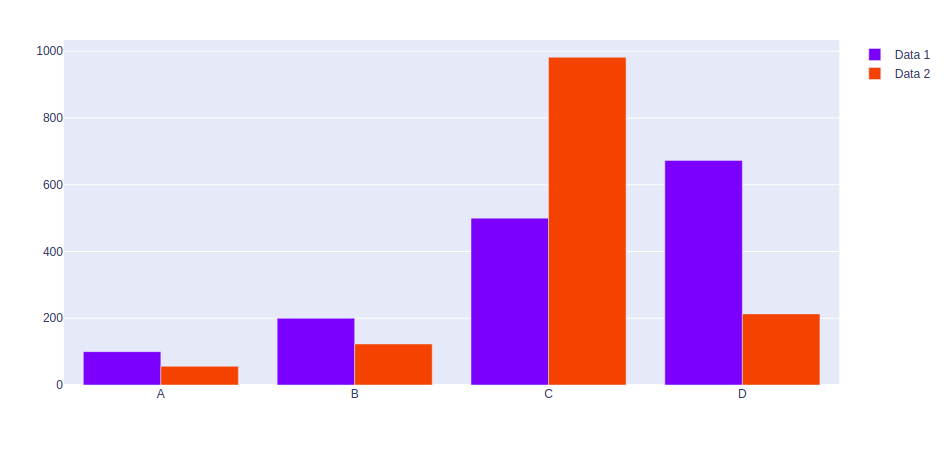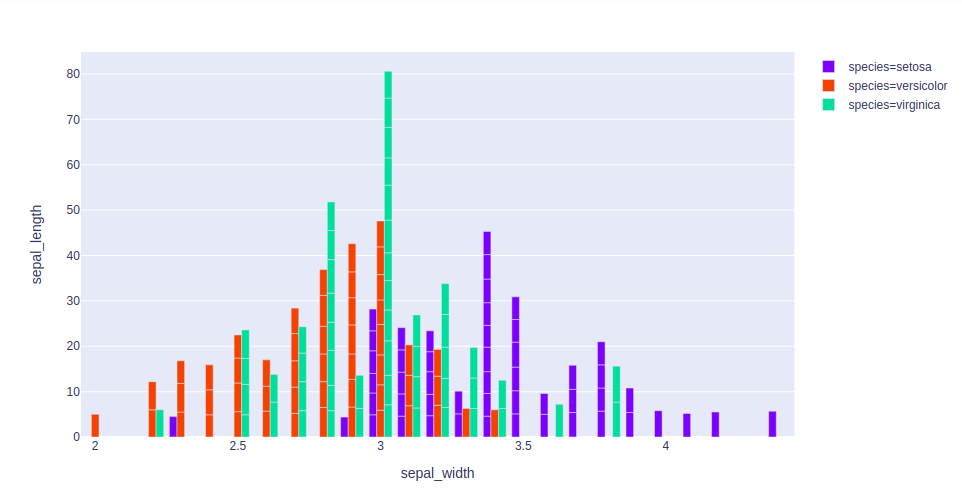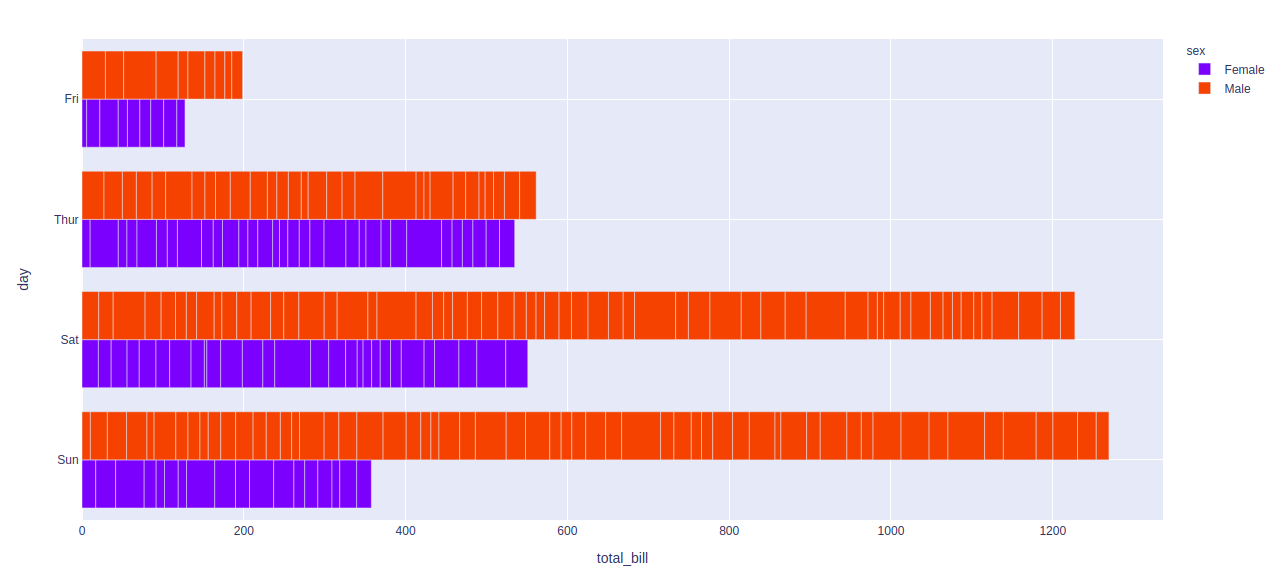How to group Bar Charts in Python-Plotly?

• Last Updated : 03 Jul, 2020

Plotly is a Python library which is used to design graphs, especially interactive graphs. It can plot various graphs and charts like histogram, barplot, boxplot, spreadplot and many more. It is mainly used in data analysis as well as financial analysis. plotly is an interactive visualization library.

Grouping Bar charts

Grouping bar charts can be used to show multiple set of data items which are been compared with a single color which is used to indicate a specific  series across all sets.

Method 1: Using graph_objects class

Example:

Python3

 import plotly.graph_objects as pximport numpy    # creating random data through randomint # function of numpy.random np.random.seed(42)  random_x= np.random.randint(1,101,100) random_y= np.random.randint(1,101,100)  x = ['A', 'B', 'C', 'D']  plot = px.Figure(data=[go.Bar(    name = 'Data 1',    x = x,    y = [100, 200, 500, 673]   ),                       go.Bar(    name = 'Data 2',    x = x,    y = [56, 123, 982, 213]   )])                   plot.show()

Output:Method 2: Using express class

Example 1: Iris dataset

Python3

 import plotly.express as px   df = px.data.iris()   fig = px.bar(df, x="sepal_width", y="sepal_length",             color="species", hover_data=['petal_width'],             barmode = 'group')   fig.show()

Output:Example 2: Tips dataset

Python3

 import plotly.express as px  df = px.data.tips()  fig = px.bar(df, x="tota_bill", y="day",              color="sex", barmode = 'group')  fig.show()

Output:My Personal Notes arrow_drop_up